Date

Today we’ll talk about unary operators, namely unary plus (+) and unary minus (-) operators.

A lot of today’s material is based on the material from the previous article, so if you need a refresher just head back to Part 7 and go over it again. Remember: repetition is the mother of all learning.

Having said that, this is what you are going to do today:

• extend the grammar to handle unary plus and unary minus operators
• add a new UnaryOp AST node class
• extend the parser to generate an AST with UnaryOp nodes
• extend the interpreter and add a new visit_UnaryOp method to interpret unary operators

Let’s get started, shall we?

So far we’ve worked with binary operators only (+, -, *, /), that is, the operators that operate on two operands.

What is a unary operator then? A unary operator is an operator that operates on one operand only.

Here are the rules for unary plus and unary minus operators:

• The unary minus (-) operator produces the negation of its numeric operand
• The unary plus (+) operator yields its numeric operand without change
• The unary operators have higher precedence than the binary operators +, -, *, and /

In the expression “+ - 3” the first ‘+’ operator represents the unary plus operation and the second ‘-‘ operator represents the unary minus operation. The expression “+ - 3” is equivalent to “+ (- (3))” which is equal to -3. One could also say that -3 in the expression is a negative integer, but in our case we treat it as a unary minus operator with 3 as its positive integer operand: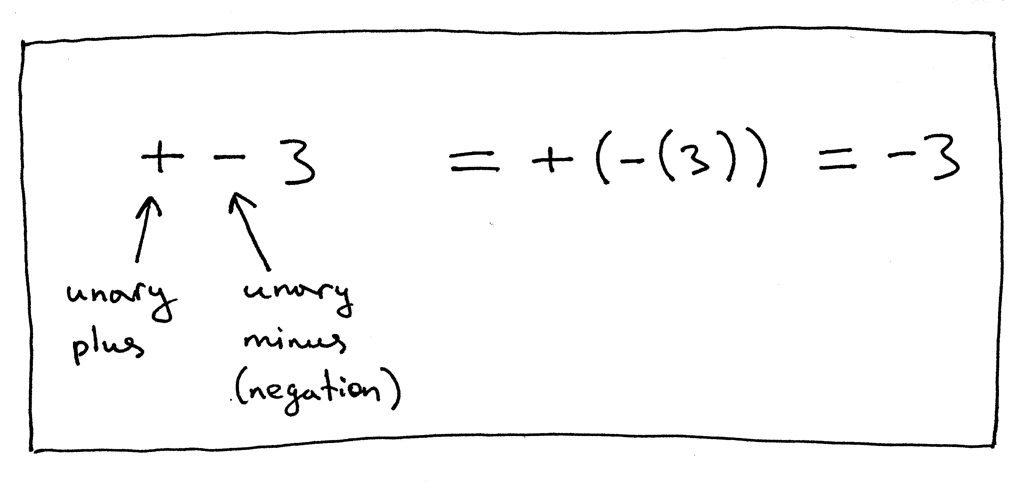Let’s take a look at another expression, “5 - - 2”: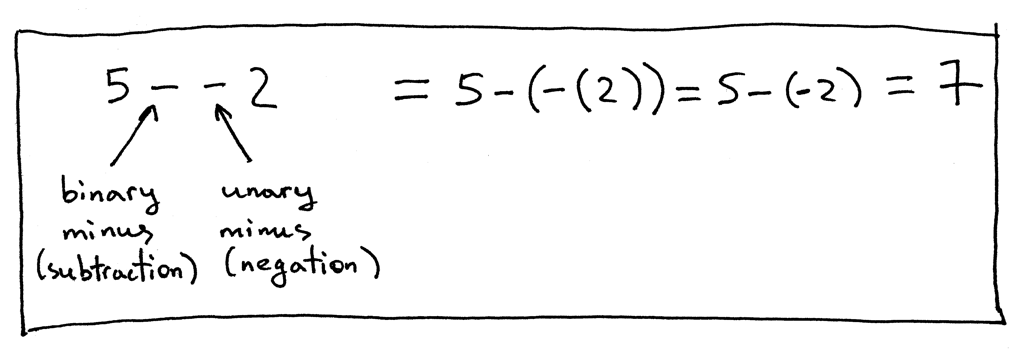In the expression “5 - - 2” the first ‘-‘ represents the binary subtraction operation and the second ‘-‘ represents the unary minus operation, the negation.

And some more examples: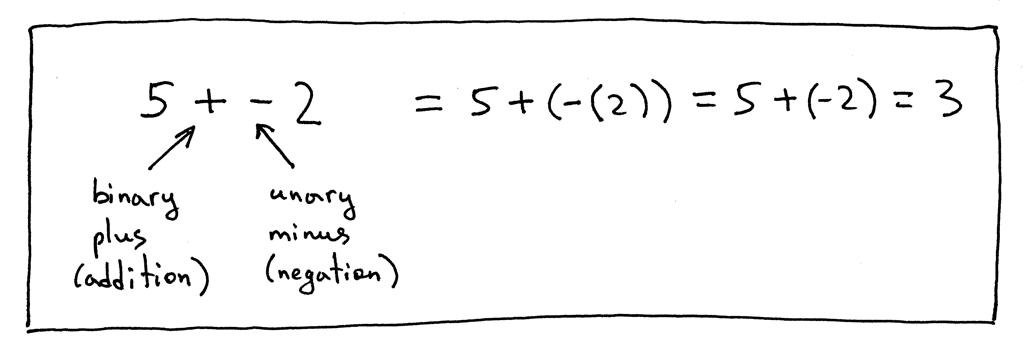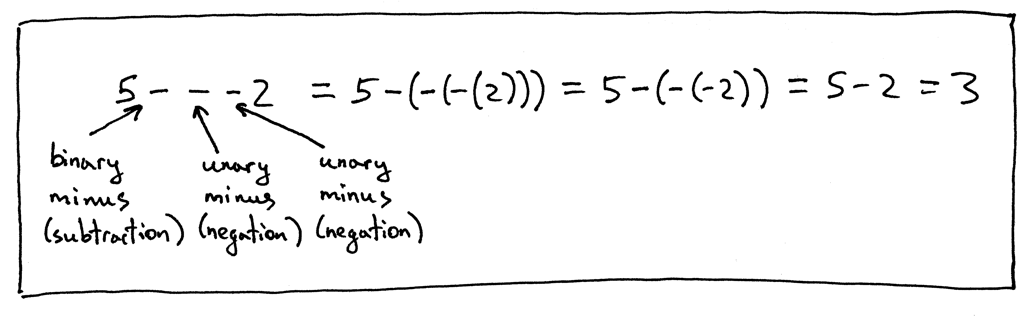Now let’s update our grammar to include unary plus and unary minus operators. We’ll modify the factor rule and add unary operators there because unary operators have higher precedence than binary +, -, * and / operators.

This is our current factor rule: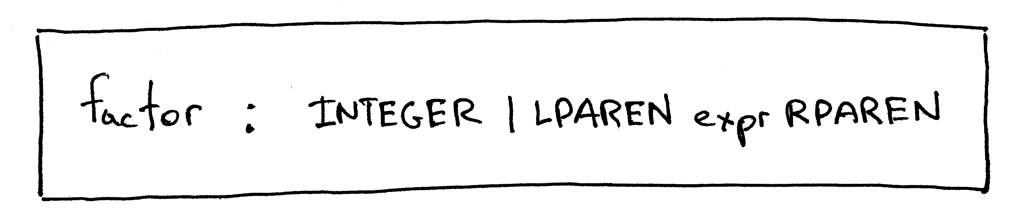And this is our updated factor rule to handle unary plus and unary minus operators: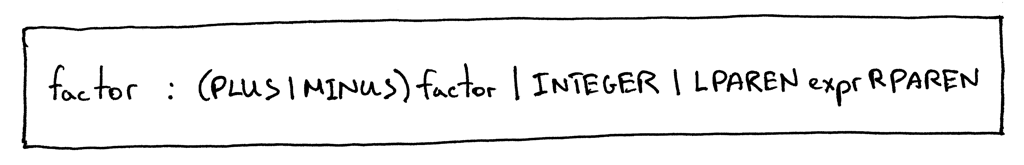As you can see, I extended the factor rule to reference itself, which allows us to derive expressions like “- - - + - 3”, a legitimate expression with a lot of unary operators.

Here is the full grammar that can now derive expressions with unary plus and unary minus operators: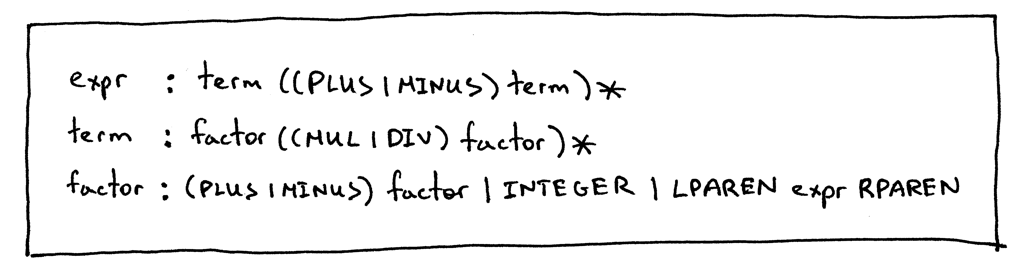The next step is to add an AST node class to represent unary operators.

This one will do:

```class UnaryOp(AST):
def __init__(self, op, expr):
self.token = self.op = op
self.expr = expr
```

The constructor takes two parameters: op, which represents the unary operator token (plus or minus) and expr, which represents an AST node.

Our updated grammar had changes to the factor rule, so that’s what we’re going to modify in our parser - the factor method. We will add code to the method to handle the “(PLUS | MINUS) factor” sub-rule:

```def factor(self):
"""factor : (PLUS | MINUS) factor | INTEGER | LPAREN expr RPAREN"""
token = self.current_token
if token.type == PLUS:
self.eat(PLUS)
node = UnaryOp(token, self.factor())
return node
elif token.type == MINUS:
self.eat(MINUS)
node = UnaryOp(token, self.factor())
return node
elif token.type == INTEGER:
self.eat(INTEGER)
return Num(token)
elif token.type == LPAREN:
self.eat(LPAREN)
node = self.expr()
self.eat(RPAREN)
return node
```

And now we need to extend the Interpreter class and add a visit_UnaryOp method to interpret unary nodes:

```def visit_UnaryOp(self, node):
op = node.op.type
if op == PLUS:
return +self.visit(node.expr)
elif op == MINUS:
return -self.visit(node.expr)
```

Onward!

Let’s manually build an AST for the expression “5 - - - 2” and pass it to our interpreter to verify that the new visit_UnaryOp method works. Here is how you can do it from the Python shell:

```>>> from spi import BinOp, UnaryOp, Num, MINUS, INTEGER, Token
>>> five_tok = Token(INTEGER, 5)
>>> two_tok = Token(INTEGER, 2)
>>> minus_tok = Token(MINUS, '-')
>>> expr_node = BinOp(
...     Num(five_tok),
...     minus_tok,
...     UnaryOp(minus_token, UnaryOp(minus_token, Num(two_tok)))
... )
>>> from spi import Interpreter
>>> inter = Interpreter(None)
>>> inter.visit(expr_node)
3
```

Visually the above AST tree looks like this: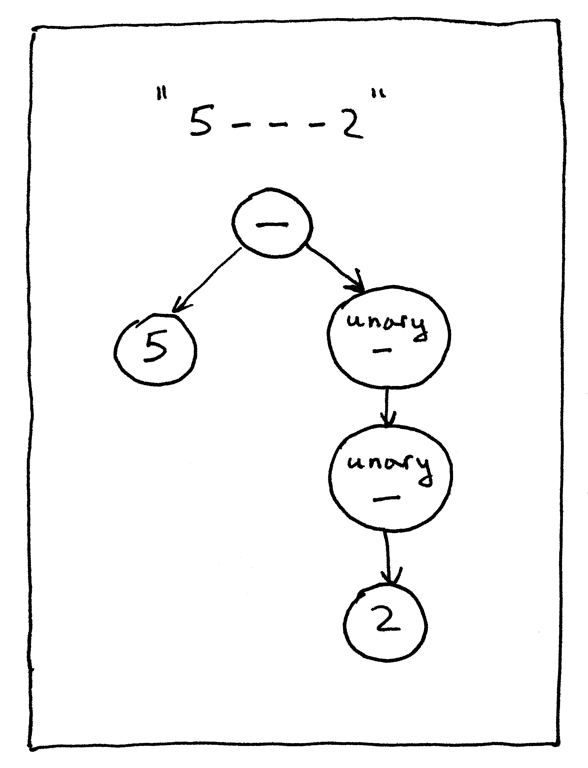Download the full source code of the interpreter for this article directly from GitHub. Try it out and see for yourself that your updated tree-based interpreter properly evaluates arithmetic expressions containing unary operators.

Here is a sample session:

```\$ python spi.py
spi> - 3
-3
spi> + 3
3
spi> 5 - - - + - 3
8
spi> 5 - - - + - (3 + 4) - +2
10
```

I also updated the genastdot.py utility to handle unary operators. Here are some of the examples of the generated AST images for expressions with unary operators:

```\$ python genastdot.py "- 3" > ast.dot && dot -Tpng -o ast.png ast.dot
```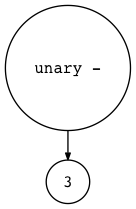```\$ python genastdot.py "+ 3" > ast.dot && dot -Tpng -o ast.png ast.dot
```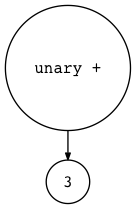```\$ python genastdot.py "5 - - - + - 3" > ast.dot && dot -Tpng -o ast.png ast.dot
```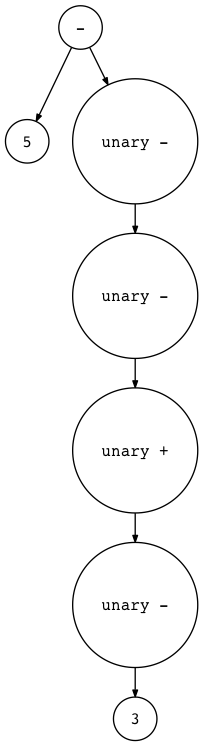```\$ python genastdot.py "5 - - - + - (3 + 4) - +2" \
> ast.dot && dot -Tpng -o ast.png ast.dot
```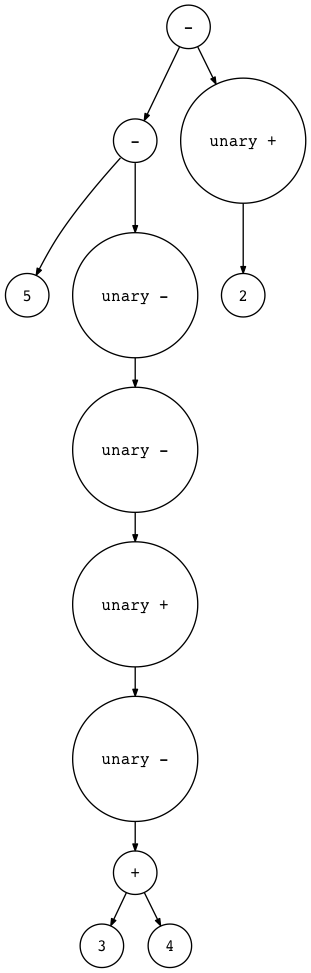And here is a new exercise for you:That’s all for today. In the next article, we’ll tackle assignment statements. Stay tuned and see you soon.

Here is a list of books I recommend that will help you in your study of interpreters and compilers:

1. Language Implementation Patterns: Create Your Own Domain-Specific and General Programming Languages (Pragmatic Programmers)2. Writing Compilers and Interpreters: A Software Engineering Approach3. Modern Compiler Implementation in Java4. Modern Compiler Design5. Compilers: Principles, Techniques, and Tools (2nd Edition)